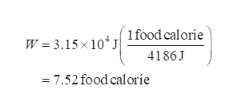# A 59.0 kg cheetah accelerates from rest to its top speed of 32.7 m/s (a)How much net work (in J) is required for the cheetah to reach its top speed? (b)One food Calorie equals 4186 J. How many Calories of net work are required for the cheetah to reach its top speed? Note: Due to inefficiencies in converting chemical energy to mechanical energy, the amount calculated here is only a fraction of the energy that must be produced by the cheetah's body.

Question
1 views

A 59.0 kg cheetah accelerates from rest to its top speed of 32.7 m/s

(a)
How much net work (in J) is required for the cheetah to reach its top speed?

(b)
One food Calorie equals 4186 J. How many Calories of net work are required for the cheetah to reach its top speed? Note: Due to inefficiencies in converting chemical energy to mechanical energy, the amount calculated here is only a fraction of the energy that must be produced by the cheetah's body.
check_circle

Step 1

a)

Work done is equal to change in kinetic energy.

Step 2

Step 3

b)

Work done in calo...help_outlineImage Transcriptionclose1 food calorie W = 3.15 x 10 J 4186J = 7.52 food calorie fullscreen

### Want to see the full answer?

See Solution

#### Want to see this answer and more?

Solutions are written by subject experts who are available 24/7. Questions are typically answered within 1 hour.*

See Solution
*Response times may vary by subject and question.
Tagged in

### Science